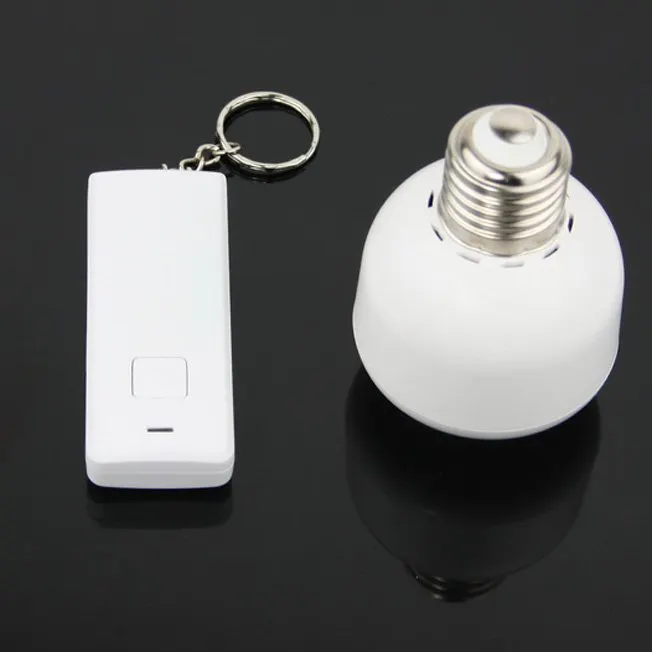# Switch on the Light - SOL

Simple project using TSOP.

BeginnerShowcase (no instructions)1 hour4,232## Things used in this project

### Hardware components

 TSOP
×1Arduino UNO
×13 mm LED: Green
×1

## Code

### Light control

Arduino
Simple code to control Lights using T.V Remote and TSOP receiver
```#include <IRremote.h>

int RECV_PIN = 11; //
int output1 = 2;
int output2 = 4;
int output3 = 6;
int itsONled[] = {0,0,0,0};

#define code1  0x4FF807F //
#define code2  0x4FF40BF //
#define code3  0x4FF20DF //

IRrecv irrecv(RECV_PIN);

decode_results results;

void setup()
{
Serial.begin(9600);   //
irrecv.enableIRIn();  //
pinMode(output1, OUTPUT);
pinMode(output2, OUTPUT);
pinMode(output3, OUTPUT);
}

void loop() {
if (irrecv.decode(&results)) {
unsigned int value = results.value;
switch(value) {
case code1:
if(itsONled == 1) {        //
digitalWrite(output1, LOW);   //
itsONled = 0;           //
} else {                      //
digitalWrite(output1, HIGH); //
itsONled = 1;          //
}
break;
case code2:
if(itsONled == 1) {
digitalWrite(output2, LOW);
itsONled = 0;
} else {
digitalWrite(output2, HIGH);
itsONled = 1;
}
break;
case code3:
if(itsONled == 1) {
digitalWrite(output3, LOW);
itsONled = 0;
} else {
digitalWrite(output3, HIGH);
itsONled = 1;
}
break;
}
Serial.println(value); // you can comment this line
irrecv.resume(); // Receive the next value
}
}
```

## Credits

### Akash Ravichandran

15 projects • 92 followers
Developer and a Learner